# Introduction to forestploter

#### 2023-09-23

Forest plots are commonly used in the medical research publications, especially in meta-analysis. And it can also be used to report the coefficients and confidence intervals (CIs) of the regression models.

There are lots of packages out there can be used to draw a forest plot. The most popular one is forestplot. Packages specialised for the meta-analysis, like meta, metafor and rmeta. Some other packages, like ggforestplot uses ggplot2 to draw a forest plot, it is not available on the CRAN yet.

The main differences of the forestploter from the other packages are:

• Focus on the forest plot.
• Treat the forest plot as a table, elements are aligned in rows and columns. User has full control on what and how to display in the forest plot contents.
• Graphical parameters are controlled with a theme.
• Allowing post-hoc plot editing.
• CIs in multiple columns and by groups.

# Basic forest plot

The layout of the forest plot is determined by the dataset provided. Please check out the other vignette if you want change the text or background, add or insert text, add borders to cells, edit color of the CI in some cells.

## Simple forest plot

The first step is to prepare a data.frame to be used as a basic layout of the forest plot. Column names of the data will be drawn as the header, and contents inside the data will be displayed in the forest plot. One or multiple blank columns without any content (blanks) should be provided to draw confidence interval. Width of the box to draw the CI is determined by the width of this column. Increase the number of space in the column to give more space to draw CI.

First we need to get the data ready to plot.

library(grid)
library(forestploter)

# Read provided sample example data
dt <- read.csv(system.file("extdata", "example_data.csv", package = "forestploter"))

# Keep needed columns
dt <- dt[,1:6]

# indent the subgroup if there is a number in the placebo column
dt$Subgroup <- ifelse(is.na(dt$Placebo),
dt$Subgroup, paste0(" ", dt$Subgroup))

# NA to blank or NA will be transformed to carachter.
dt$Treatment <- ifelse(is.na(dt$Treatment), "", dt$Treatment) dt$Placebo <- ifelse(is.na(dt$Placebo), "", dt$Placebo)
dt$se <- (log(dt$hi) - log(dt$est))/1.96 # Add blank column for the forest plot to display CI. # Adjust the column width with space, increase number of space below # to have a larger area to draw the CI. dt$  <- paste(rep(" ", 20), collapse = " ")

# Create confidence interval column to display
dt$HR (95% CI) <- ifelse(is.na(dt$se), "",
sprintf("%.2f (%.2f to %.2f)",
dt$est, dt$low, dt$hi)) head(dt) #> Subgroup Treatment Placebo est low hi se #> 1 All Patients 781 780 1.869694 0.13245636 3.606932 0.3352463 #> 2 Sex NA NA NA NA #> 3 Male 535 548 1.449472 0.06834426 2.830600 0.3414741 #> 4 Female 246 232 2.275120 0.50768005 4.042560 0.2932884 #> 5 Age NA NA NA NA #> 6 <65 yr 297 333 1.509242 0.67029394 2.348190 0.2255292 #> HR (95% CI) #> 1 1.87 (0.13 to 3.61) #> 2 #> 3 1.45 (0.07 to 2.83) #> 4 2.28 (0.51 to 4.04) #> 5 #> 6 1.51 (0.67 to 2.35) The data we have above will be used as basic layout of the forest plot. The example below shows how to draw a simple forest plot. A footnote was added as a demonstration. p <- forest(dt[,c(1:3, 8:9)], est = dt$est,
lower = dt$low, upper = dt$hi,
sizes = dt$se, ci_column = 4, ref_line = 1, arrow_lab = c("Placebo Better", "Treatment Better"), xlim = c(0, 4), ticks_at = c(0.5, 1, 2, 3), footnote = "This is the demo data. Please feel free to change\nanything you want.") # Print plot plot(p)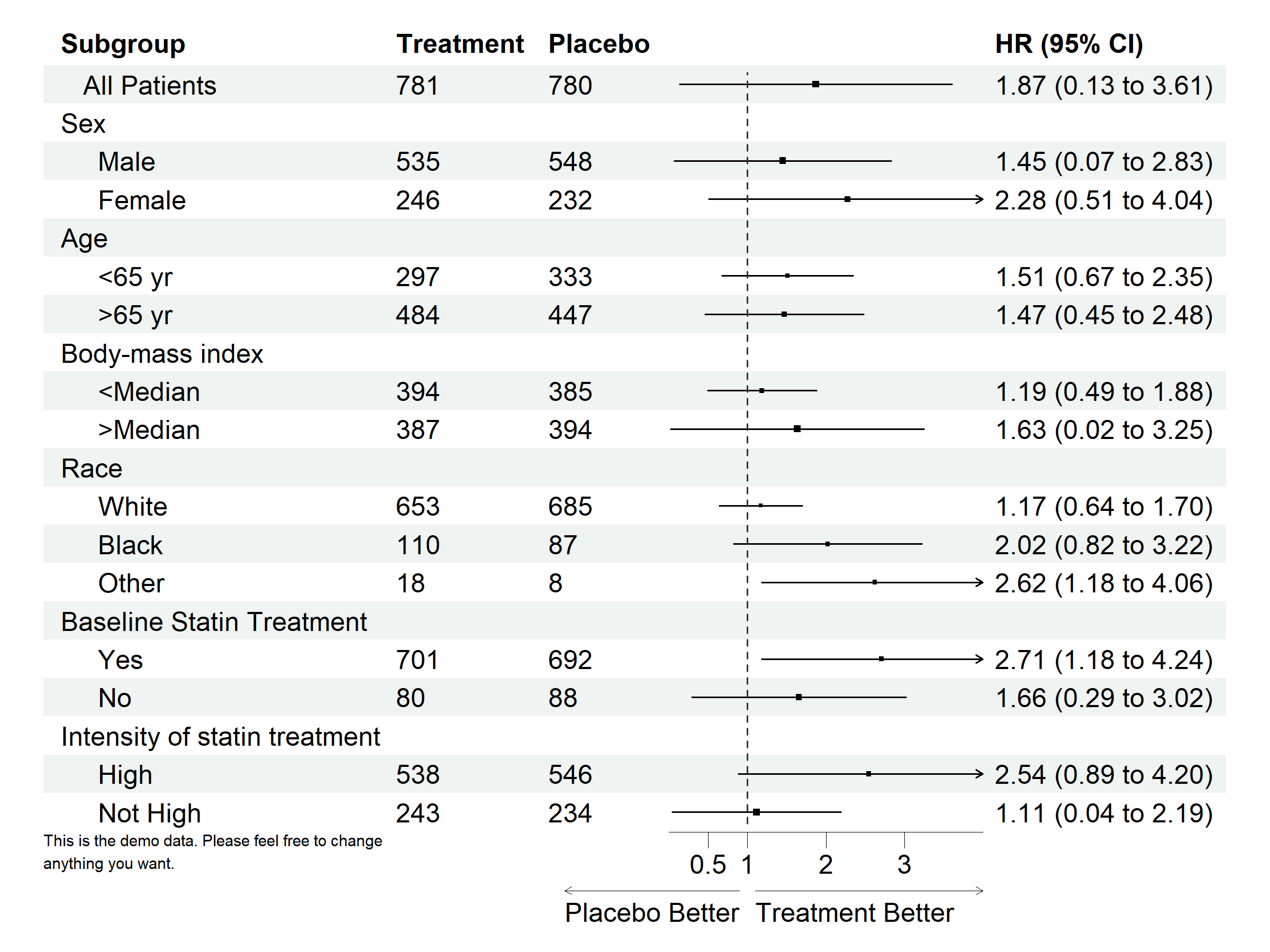## Change theme Now we will use the same data above, and add a summary point. We also want to change the graphical parameters for confidence interval and other parts of the plot. Theme of the forest plot can be adjusted with forest_theme function, check out the manual for more details. dt_tmp <- rbind(dt[-1, ], dt[1, ]) dt_tmp[nrow(dt_tmp), 1] <- "Overall" dt_tmp <- dt_tmp[1:11, ] # Define theme tm <- forest_theme(base_size = 10, # Confidence interval point shape, line type/color/width ci_pch = 15, ci_col = "#762a83", ci_fill = "black", ci_alpha = 0.8, ci_lty = 1, ci_lwd = 1.5, ci_Theight = 0.2, # Set an T end at the end of CI # Reference line width/type/color refline_lwd = 1, refline_lty = "dashed", refline_col = "grey20", # Vertical line width/type/color vertline_lwd = 1, vertline_lty = "dashed", vertline_col = "grey20", # Change summary color for filling and borders summary_fill = "#4575b4", summary_col = "#4575b4", # Footnote font size/face/color footnote_cex = 0.6, footnote_fontface = "italic", footnote_col = "blue") pt <- forest(dt_tmp[,c(1:3, 8:9)], est = dt_tmp$est,
lower = dt_tmp$low, upper = dt_tmp$hi,
sizes = dt_tmpse, is_summary = c(rep(FALSE, nrow(dt_tmp)-1), TRUE), ci_column = 4, ref_line = 1, arrow_lab = c("Placebo Better", "Treatment Better"), xlim = c(0, 4), ticks_at = c(0.5, 1, 2, 3), footnote = "This is the demo data. Please feel free to change\nanything you want.", theme = tm) # Print plot plot(pt)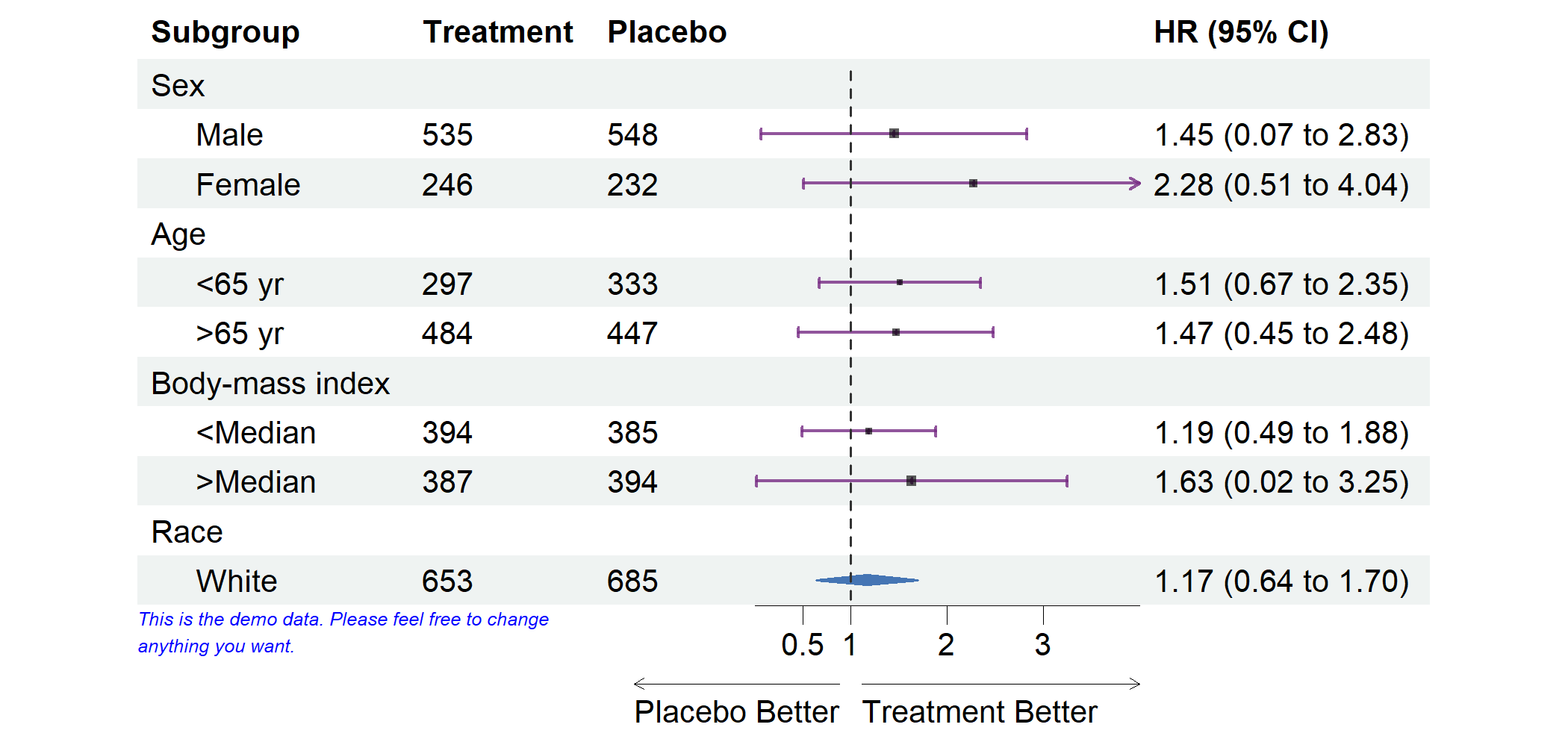## Text justification and background with theme By default, all cells are left aligned. But it is possible to justify any cells in the forest plot by setting parameters in forest_theme. Set core=list(fg_params=list(hjust=0, x=0)) to left align content, and rowhead=list(fg_params=list(hjust=0.5, x=0.5) to center header. Set hjust=1 and x=0.9 to right align text. You can also change the justification of text by with edit_plot, see details in another vignette. Same rule apply to change the background color by setting core=list(bg_params=list(fill = c("#edf8e9", "#c7e9c0", "#a1d99b"))). Change settings in core if you want to change graphical parameters of contents, colhead for header. Change settings in fg_params to modify the text, see parameters for textGrob() in grid package. Change bg_params to modify settings for background graphical parameters, see gpar() in grid package. You should pass parameters as a list. More details can be found here. Provide a single value if you want cells have the same justification or vector for each cells. As you can notice that the second example justify text by row using the provided vector, and the vector will be recycled. dt <- dt[1:4, ] # Header center and content right tm <- forest_theme(core=list(fg_params=list(hjust = 1, x = 0.9), bg_params=list(fill = c("#edf8e9", "#c7e9c0", "#a1d99b"))), colhead=list(fg_params=list(hjust=0.5, x=0.5))) p <- forest(dt[,c(1:3, 8:9)], est = dtest,
lower = dt$low, upper = dt$hi,
sizes = dt$se, ci_column = 4, title = "Header center content right", theme = tm) # Print plot plot(p)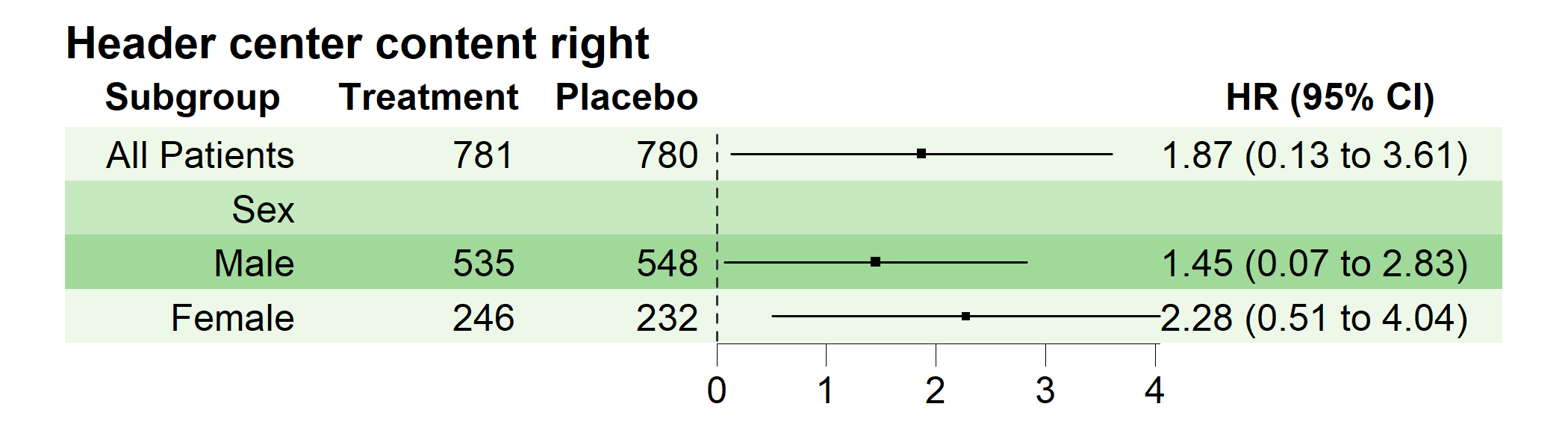# Mixed justification tm <- forest_theme(core=list(fg_params=list(hjust=c(1, 0, 0, 0.5), x=c(0.9, 0.1, 0, 0.5)), bg_params=list(fill = c("#f6eff7", "#d0d1e6", "#a6bddb", "#67a9cf"))), colhead=list(fg_params=list(hjust=c(1, 0, 0, 0, 0.5), x=c(0.9, 0.1, 0, 0, 0.5)))) p <- forest(dt[,c(1:3, 8:9)], est = dt$est,
lower = dt$low, upper = dt$hi,
sizes = dt$se, ci_column = 4, title = "Mixed justification", theme = tm) plot(p)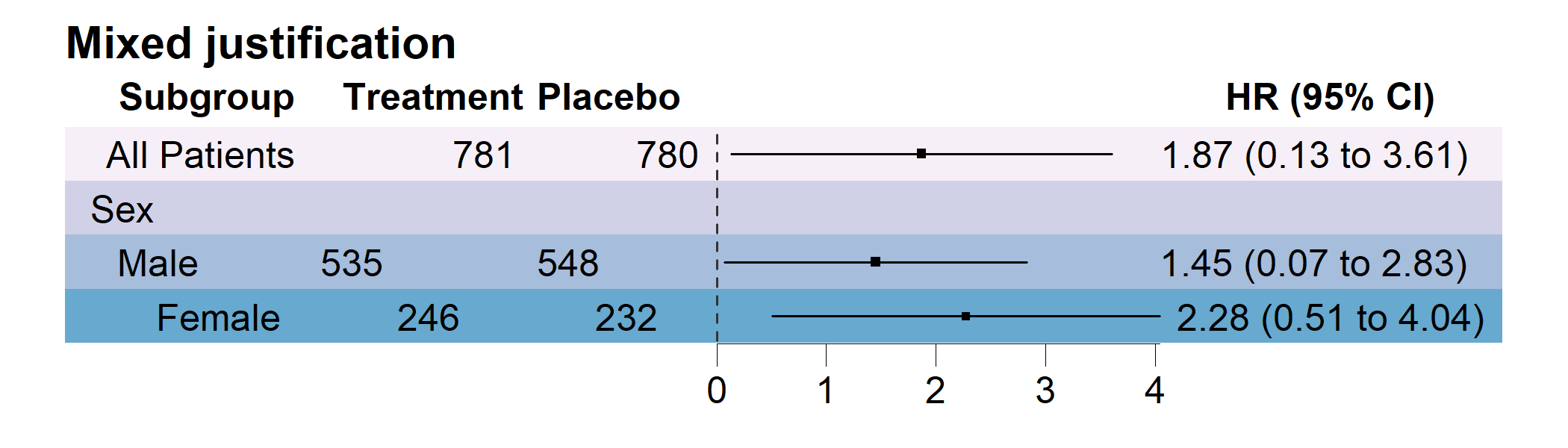# Multiple CI columns Sometimes one may want to have multiple CI columns, each column may represent different outcome. If this is the case, one only needs to provide a vector of the position of the columns to be drawn in the data. If the number of columns provided to draw the CI columns are same as number of est, one CI will be drawn into each CI columns. If the number of columns provided is less than number of est, the extra est will be considered as a group and will be drawn to the CI columns sequentially. In the latter case, group number equals to number of est divided by number of ci_column and multiple columns will be drawn into one cell. As seen in the example below, the CI will be drawn in the column 3 and 5. The first and second elements in est, lower and upper will be drawn in column 3 and column 5. In a multiple groups example, two or more CI in one cell. The solution is simple, provide all the values sequentially to est, lower and upper. Which means, the first n elements in the est, lower and upper are considered as same group, same for next n elements. The n is determined by the number of ci_column. As it is shown in the example below, est_gp1 and est_gp2 will be drawn in column 3 and column 5, considered as group 1. The est_gp3 and est_gp4 will be drawn in column 3 and column 5, considered as group 2. This is an example of multiple CI columns and groups: dt <- read.csv(system.file("extdata", "example_data.csv", package = "forestploter")) dt <- dt[1:7, ] # indent the subgroup if there is a number in the placebo column dt$Subgroup <- ifelse(is.na(dt$Placebo), dt$Subgroup,
paste0("   ", dt$Subgroup)) # NA to blank or NA will be transformed to carachter. dt$n1 <- ifelse(is.na(dt$Treatment), "", dt$Treatment)
dt$n2 <- ifelse(is.na(dt$Placebo), "", dt$Placebo) # Add two blank column for CI dt$CVD outcome <- paste(rep(" ", 20), collapse = " ")
dt$COPD outcome <- paste(rep(" ", 20), collapse = " ") # Generate point estimation and 95% CI. Paste two CIs together and separate by line break. dt$ci1 <- paste(sprintf("%.1f (%.1f, %.1f)", dt$est_gp1, dt$low_gp1, dt$hi_gp1), sprintf("%.1f (%.1f, %.1f)", dt$est_gp3, dt$low_gp3, dt$hi_gp3),
sep = "\n")
dt$ci1[grepl("NA", dt$ci1)] <- "" # Any NA to blank

dt$ci2 <- paste(sprintf("%.1f (%.1f, %.1f)", dt$est_gp2, dt$low_gp2, dt$hi_gp2),
sprintf("%.1f (%.1f, %.1f)", dt$est_gp4, dt$low_gp4, dt$hi_gp4), sep = "\n") dt$ci2[grepl("NA", dt$ci2)] <- "" # Set-up theme tm <- forest_theme(base_size = 10, refline_lty = "solid", ci_pch = c(15, 18), ci_col = c("#377eb8", "#4daf4a"), footnote_col = "blue", legend_name = "Group", legend_value = c("Trt 1", "Trt 2"), vertline_lty = c("dashed", "dotted"), vertline_col = c("#d6604d", "#bababa"), # Table cell padding, width 4 and heights 3 core = list(padding = unit(c(4, 3), "mm"))) p <- forest(dt[,c(1, 19, 23, 21, 20, 24, 22)], est = list(dt$est_gp1,
dt$est_gp2, dt$est_gp3,
dt$est_gp4), lower = list(dt$low_gp1,
dt$low_gp2, dt$low_gp3,
dt$low_gp4), upper = list(dt$hi_gp1,
dt$hi_gp2, dt$hi_gp3,
dthi_gp4), ci_column = c(4, 7), ref_line = 1, vert_line = c(0.5, 2), nudge_y = 0.4, theme = tm) plot(p)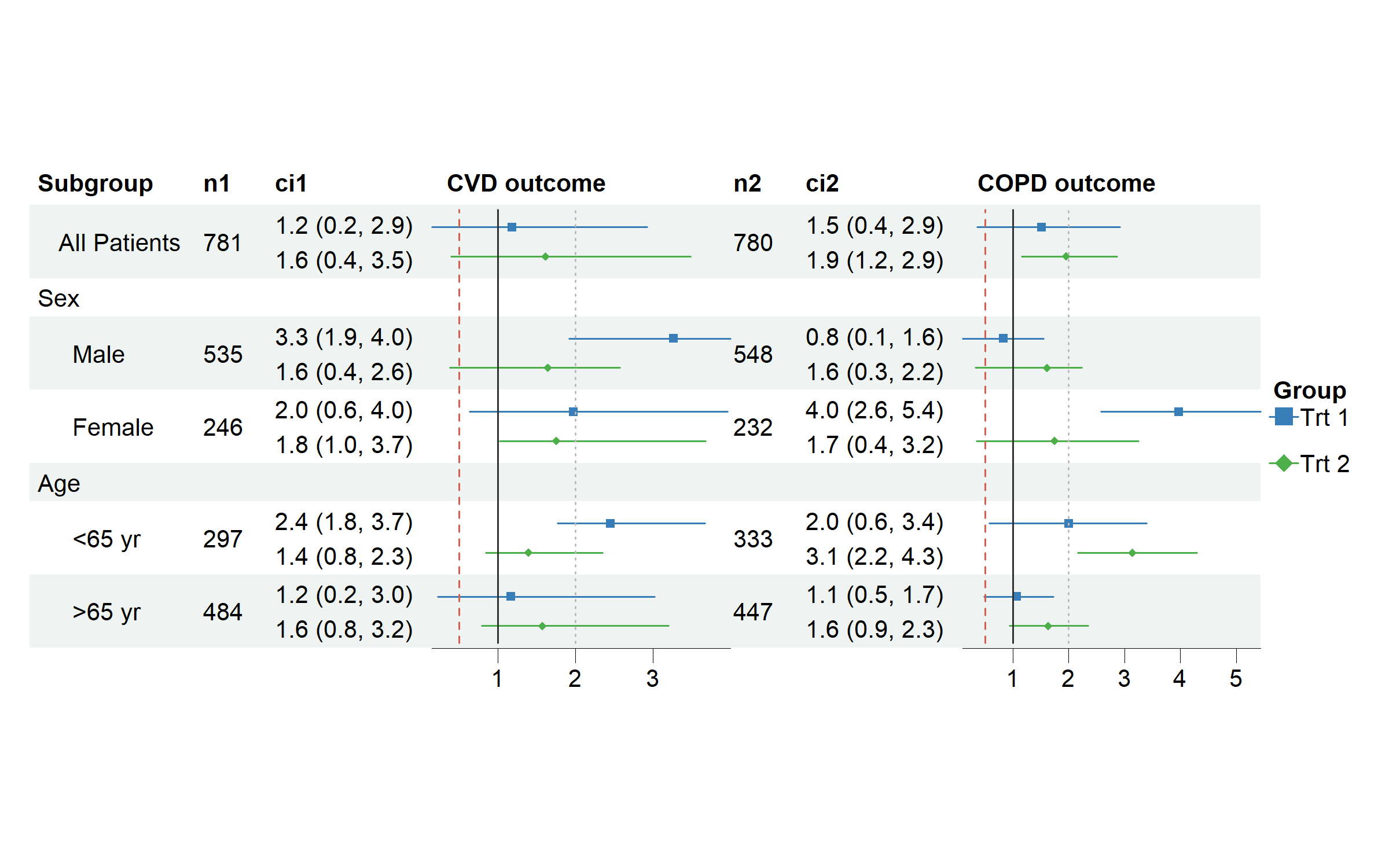It is obvious that the forest use whatever you provided as the skeleton of the forest plot. You can use your imagination and put whatever you want in a cell, including linebreaks. Please checkout the other vignette to modify the alignment of the text. # Different parameters for different CI columns If the desired forest plot is multiple column, some may want to have different settings for different columns. For example, different CI column has different xlim, x-axis ticks, x-axis labels, x_trans, reference line, vertical line or arrow labels. This can be easily done by providing a list or vector. Provide a list for xlim, vert_line, arrow_lab and ticks_at, atomic vector for xlab, x_trans and ref_line. See the example below.  dtHR (95% CI) <- ifelse(is.na(dt$est_gp1), "", sprintf("%.2f (%.2f to %.2f)", dt$est_gp1, dt$low_gp1, dt$hi_gp1))
dt$Beta (95% CI) <- ifelse(is.na(dt$est_gp2), "",
sprintf("%.2f (%.2f to %.2f)",
dt$est_gp2, dt$low_gp2, dt$hi_gp2)) tm <- forest_theme(arrow_type = "closed", arrow_label_just = "end") p <- forest(dt[,c(1, 21, 23, 22, 24)], est = list(dt$est_gp1,
dt$est_gp2), lower = list(dt$low_gp1,
dt$low_gp2), upper = list(dt$hi_gp1,
dt$hi_gp2), ci_column = c(2, 4), ref_line = c(1, 0), vert_line = list(c(0.3, 1.4), c(0.6, 2)), x_trans = c("log", "none"), arrow_lab = list(c("L1", "R1"), c("L2", "R2")), xlim = list(c(0, 3), c(-1, 3)), ticks_at = list(c(0.1, 0.5, 1, 2.5), c(-1, 0, 2)), xlab = c("OR", "Beta"), nudge_y = 0.2, theme = tm) plot(p)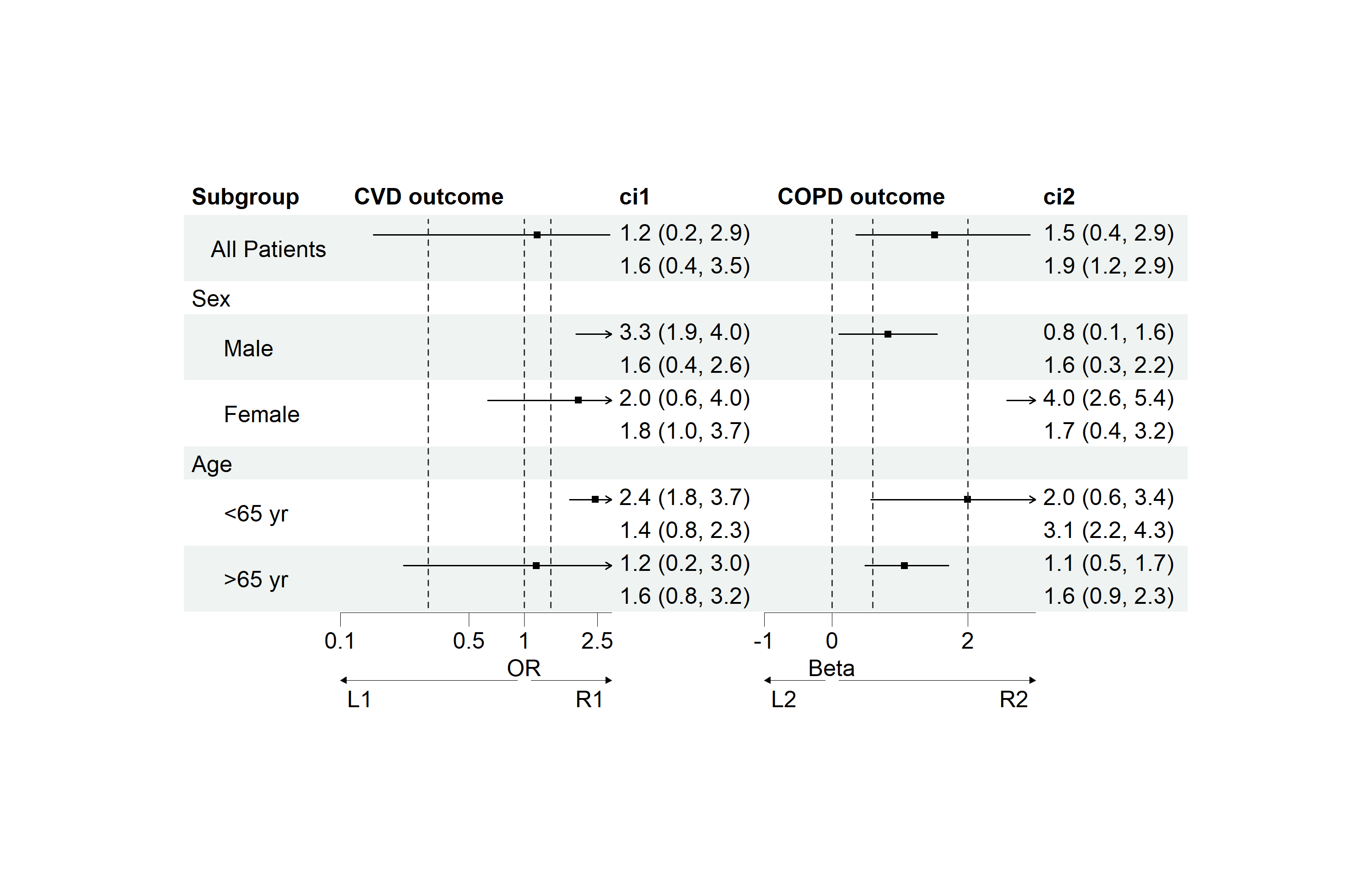# Custom CIs It is possible to pass custom CI drawing function to forest. The fn_ci accepts CI drawing function for normal confidence interval and fn_summary for summary CI. Other parameters for the those functions can be passed via forest. If you need to row values as est and lower to those functions, you need to define the name of the parameters you have passed via index_args. This is an advanced technique and not the purpose of this vignette to show how to create a function to draw CI, but you can find some tutorial here if you are interested. Below is an example of the usage for a box plot CI with the built in make_boxplot function. # Function to calculate Box plot values box_func <- function(x){ iqr <- IQR(x) q3 <- quantile(x, probs = c(0.25, 0.5, 0.75), names = FALSE) c("min" = q3 - 1.5*iqr, "q1" = q3, "med" = q3, "q3" = q3, "max" = q3 + 1.5*iqr) } # Prepare data val <- split(ToothGrowth$len, list(ToothGrowth$supp, ToothGrowth$dose))
val <- lapply(val, box_func)

dat <- do.call(rbind, val)
dat <- data.frame(Dose = row.names(dat),
dat, row.names = NULL)

dat$Box <- paste(rep(" ", 20), collapse = " ") # Draw single group box plot tm <- forest_theme(ci_Theight = 0.2) p <- forest(dat[,c(1, 7)], est = dat$med,
lower = dat$min, upper = dat$max,
# sizes = sizes,
fn_ci = make_boxplot,
ci_column = 2,
lowhinge = dat$q1, uphinge = dat$q3,
hinge_height = 0.2,
# values of the lowhinge and uphinge will be used as row values
index_args = c("lowhinge", "uphinge"),
gp_box = gpar(fill = "black", alpha = 0.4),
theme = tm
)
p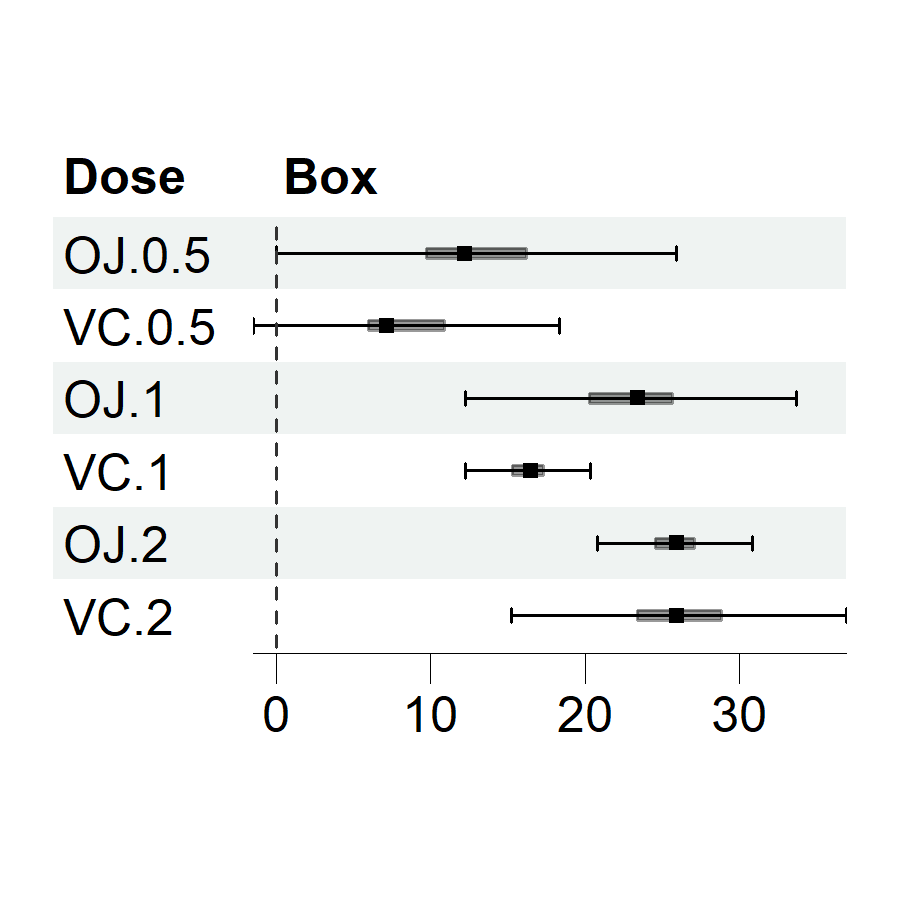# Saving plot

One can use the base method or use ggsave function to save plot. For the ggsave function, please don’t ignore the plot parameter. The width and height should be tuned to get a desired plot. You can also set autofit=TRUE in the print or plot function to auto fit the plot, but this may change not be compact as it should be.

# Base method
png('rplot.png', res = 300, width = 7.5, height = 7.5, units = "in")
p
dev.off()

# ggsave function
ggplot2::ggsave(filename = "rplot.png", plot = p,
dpi = 300,
width = 7.5, height = 7.5, units = "in")

Or you can get the width and height of the forestplot with get_wh, and use this width and height for saving.

# Get width and height
p_wh <- get_wh(plot = p, unit = "in")
png('rplot.png', res = 300, width = p_wh, height = p_wh, units = "in")
p
dev.off()

# Or get scale
get_scale <- function(plot,
width_wanted,
height_wanted,
unit = "in"){
h <- convertHeight(sum(plot$heights), unit, TRUE) w <- convertWidth(sum(plot$widths), unit, TRUE)
max(c(w/width_wanted,  h/height_wanted))
}
p_sc <- get_scale(plot = p, width_wanted = 6, height_wanted = 4, unit = "in")
ggplot2::ggsave(filename = "rplot.png",
plot = p,
dpi = 300,
width = 6,
height = 4,
units = "in",
scale = p_sc)

# FAQs

A: I have to admit that vignettes were not well written, but you should be able to get the idea if you looked the vignette carefully and checked the examples. Increase the widths by having more blank space in the column that the CI to be drawn. Please checkout the first example on how to do this.

Q: Can I modify the width and height of each row and columns?

A: Yes, although the content in of the data decide the heights and widths of the rows and columns, you can also modify these after you have finished plotting. See this here for details. You can also use core = list(padding = unit(c(4, 3), "mm")) in the forest_theme to add some padding to width and height of each cell.

Q: How should I use weight for sizes?

A: The forest function will not transform the size thus it will be used as it is. If you want to weight the size on your own, checkout here for some options

Q: How can I plot grouped forestplot?

A: You can leave few blank lines to indicate the group break. You can also use arrangeGrob from gridExtra package to combine two or more forestplot.